# DSP 学习笔记 |（四）Xtensa ISA 笔记

## 一、 XCC 编译器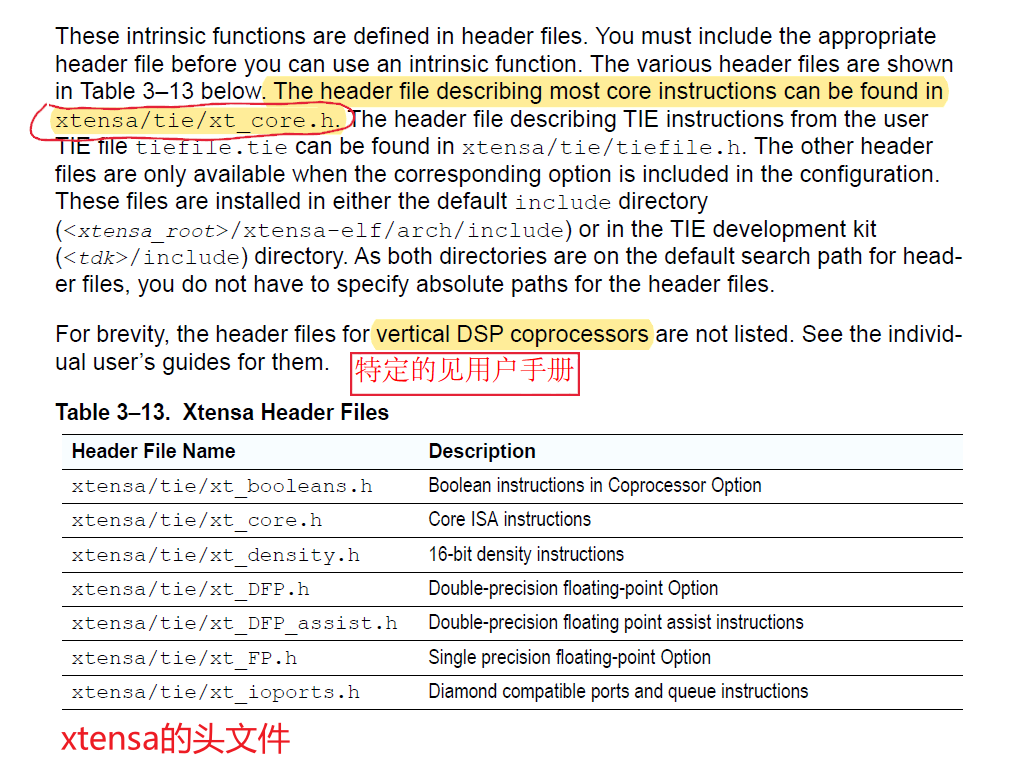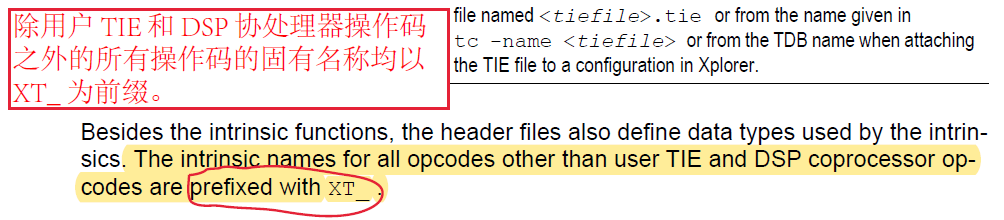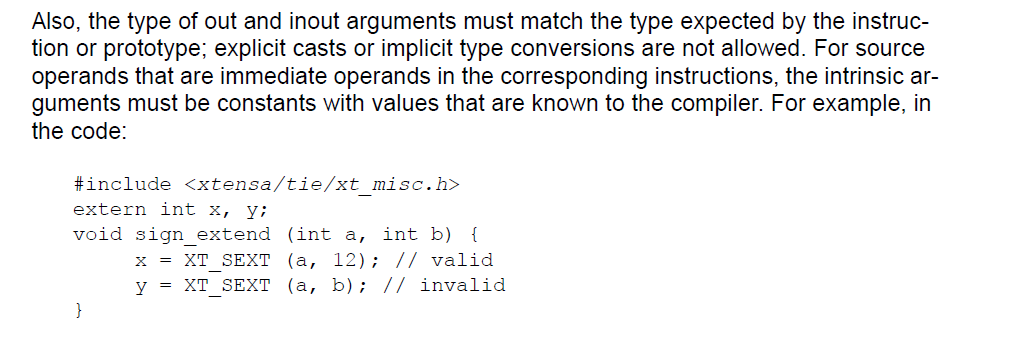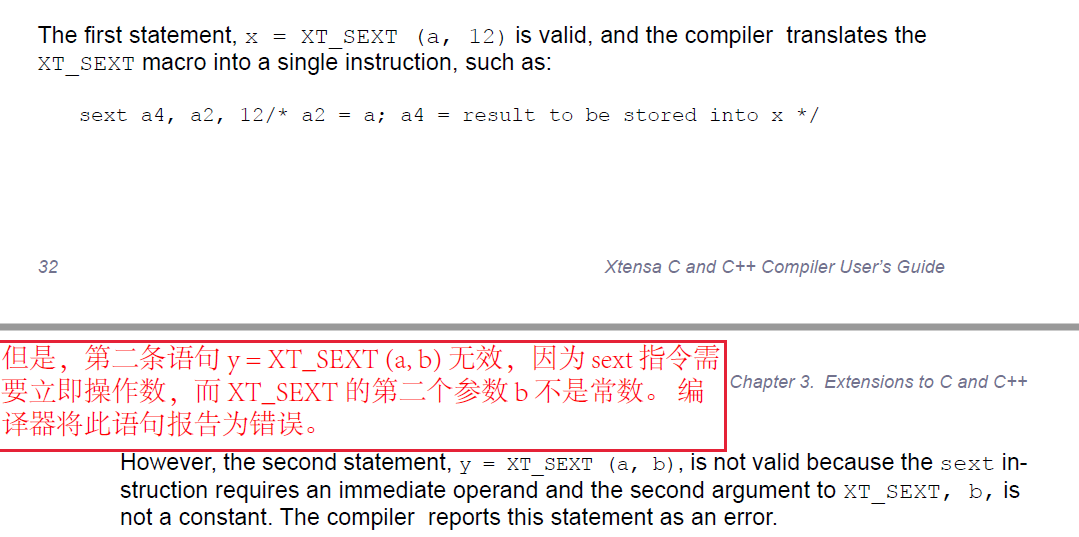## 二、 Candence 处理器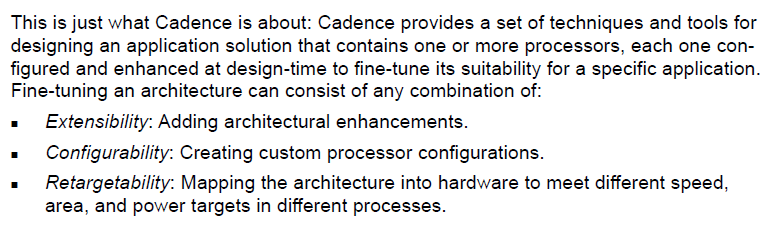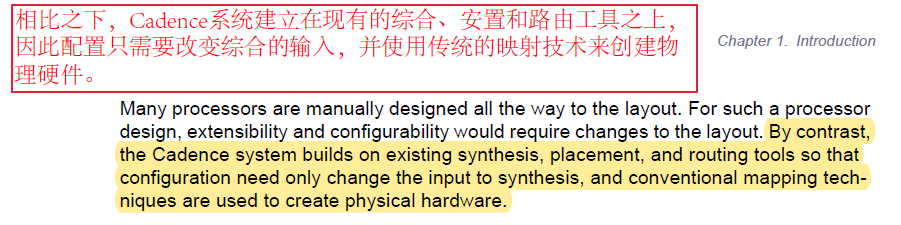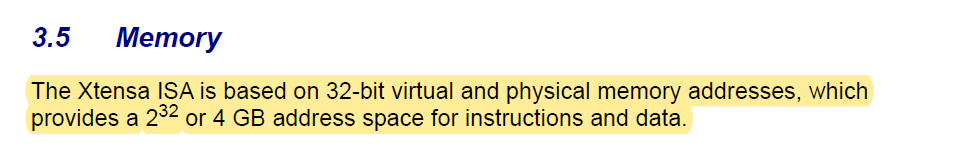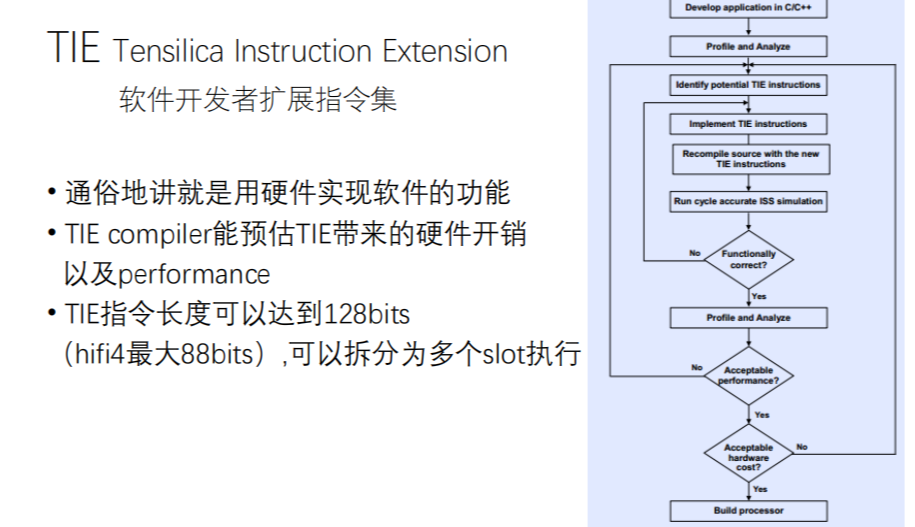## 三、 语法

### 3.1 数据类型

1. `ae_int32x2`
由两个 32 位的数据类型组成一个 64 位的数据，8 字节对齐。如果使用 int 类型强制转换，那么在内存分布上，将是两个相同的 32 位数据，举例如下：

``````void test()
{
int mem1 = 0x1234;
int mem2 = 0x5678;
ae_int32x2 p = mem1;

int *p1 = (int *)&p;
printf("(int)p = 0x%0x\n",(int)p);
printf("*(p1) = 0x%0x\n",*(p1));
printf("*(p1+1) = 0x%0x\n",*(p1+1));

ae_int32x2 p2 = AE_MOVDA32X2(mem1, mem2);
int *p3 = (int *)&p2;
printf("p3=0x%0x,*(p3) = 0x%0x\n",p3,*(p3));
printf("p3+1=0x%0x,*(p3+1) = 0x%0x\n",p3+1,*(p3+1));
}

输出：

(int)p = 0x1234
*(p1) = 0x1234
*(p1+1) = 0x1234
p3=0x2ffffeb8,*(p3) = 0x1234
p3+1=0x2ffffebc,*(p3+1) = 0x5678

分析：AE_MOVDA32X2作用是将两个32位数拼成一个64位数。``````

## 四、 基础的 SIMD 编程

SIMD ：Single Instruction Multiple Data，单指令流多数据流，是一种采用一个控制器来控制多个处理器，同时对一组数据（又称“数据向量”）中的每一个分别执行相同的操作从而实现空间上的并行性的技术。微处理器 中，单指令流多数据流技术则是一个 控制器 控制多个平行的 处理微元.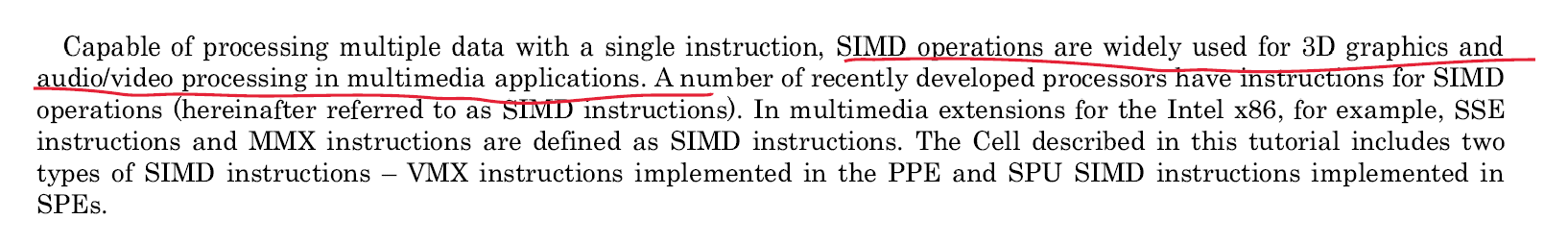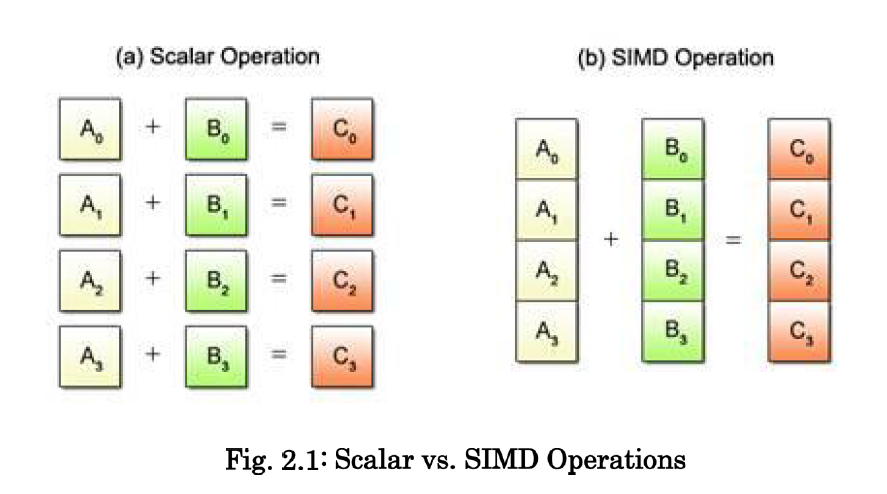SIMD 操纵不能以不同的形式处理多数据，如下图所示。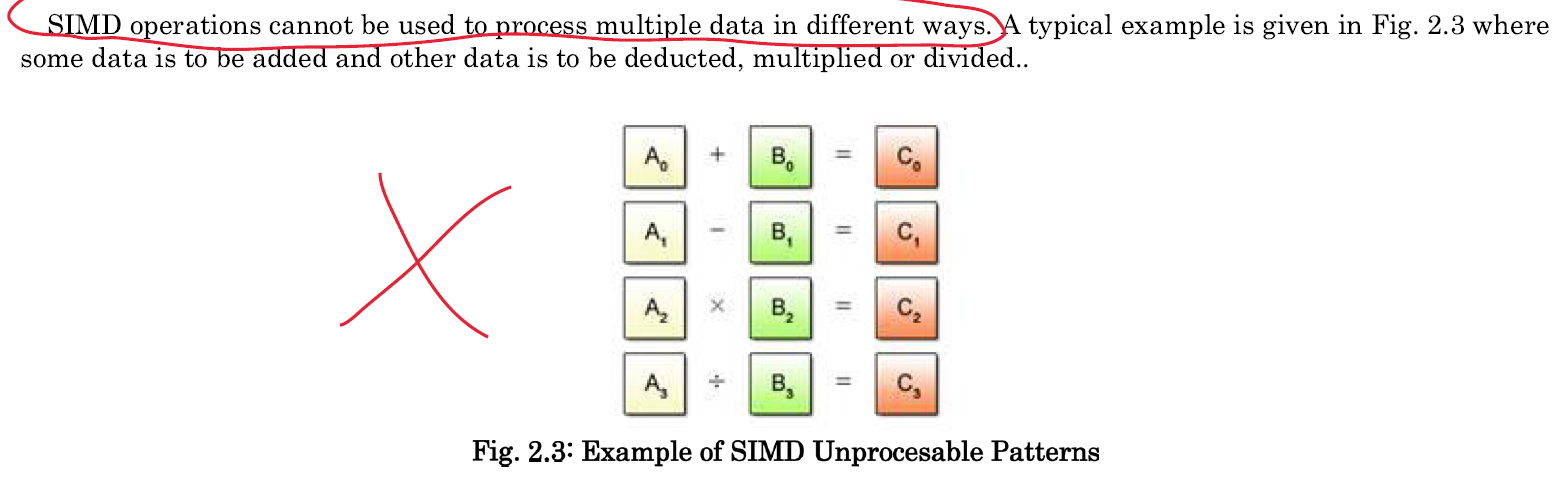### 4.1 矢量类型

C 语言常用的数据类型例如 `char``float` 被称为标量类型。在 SIMD 运算中的数据类型被称作矢量类型。每个矢量类型都有其相应的标量类型。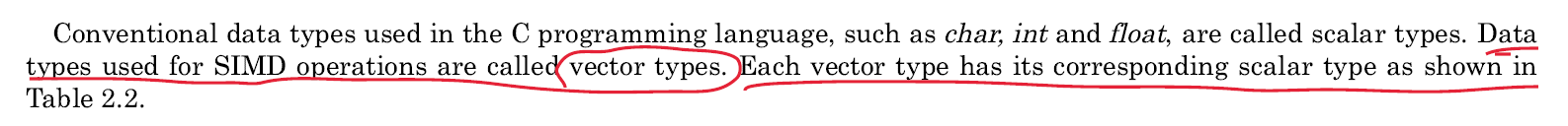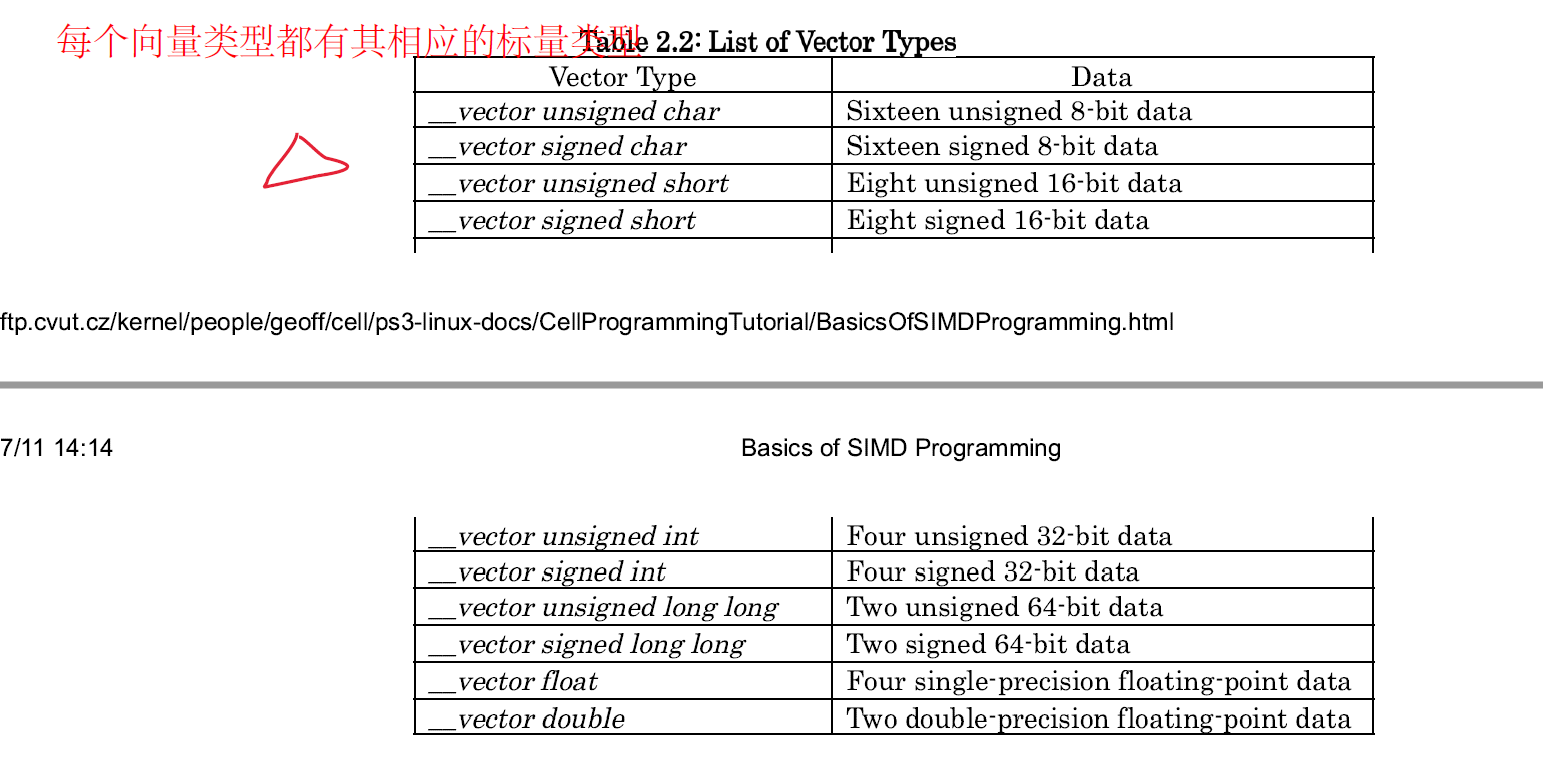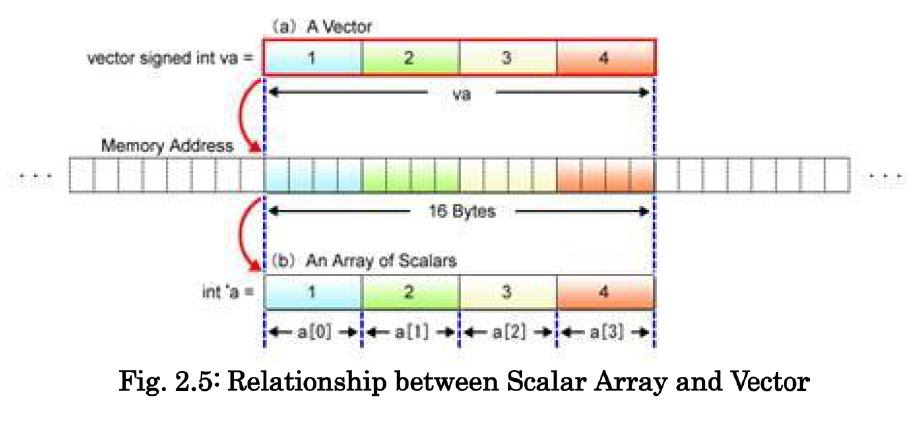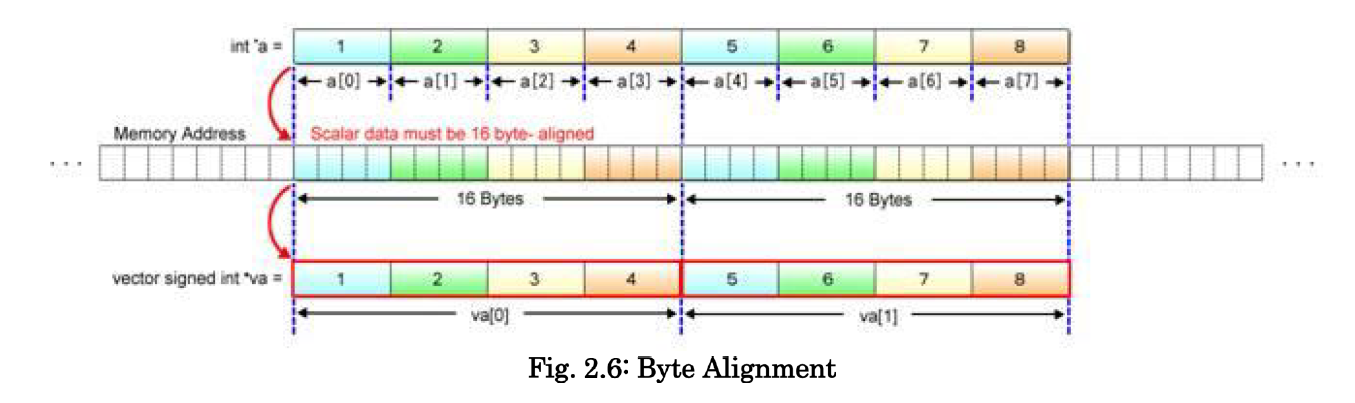### 4.2 基础的 SIMD 运算

1. 以加法为例，介绍标量运算与 SIMD 运算

a. 标量运算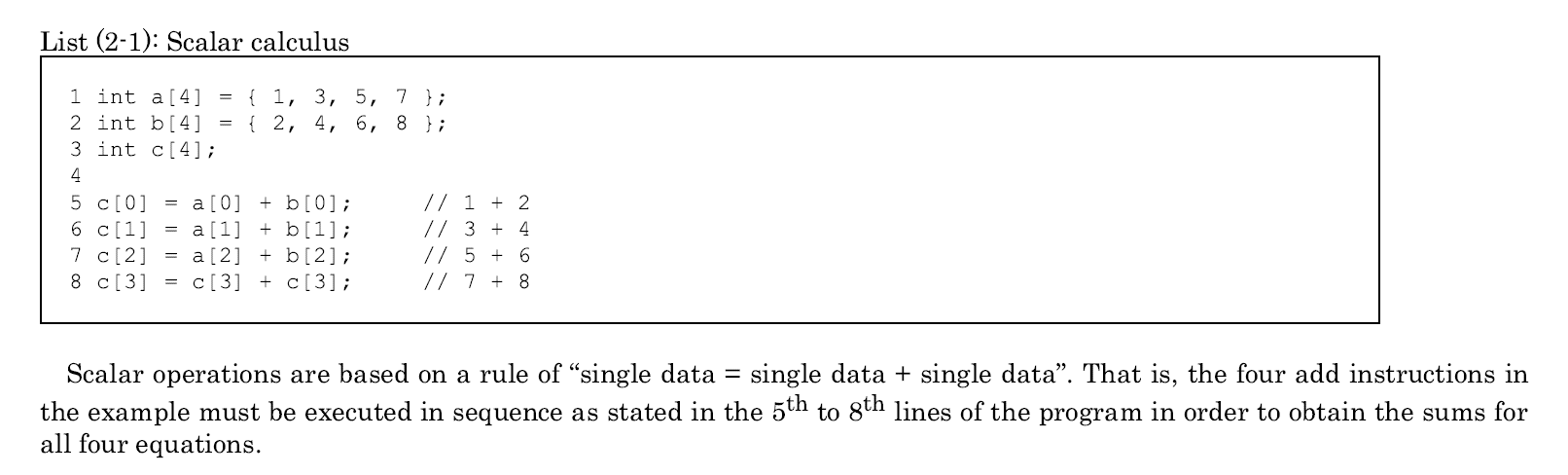b. SIMD 运算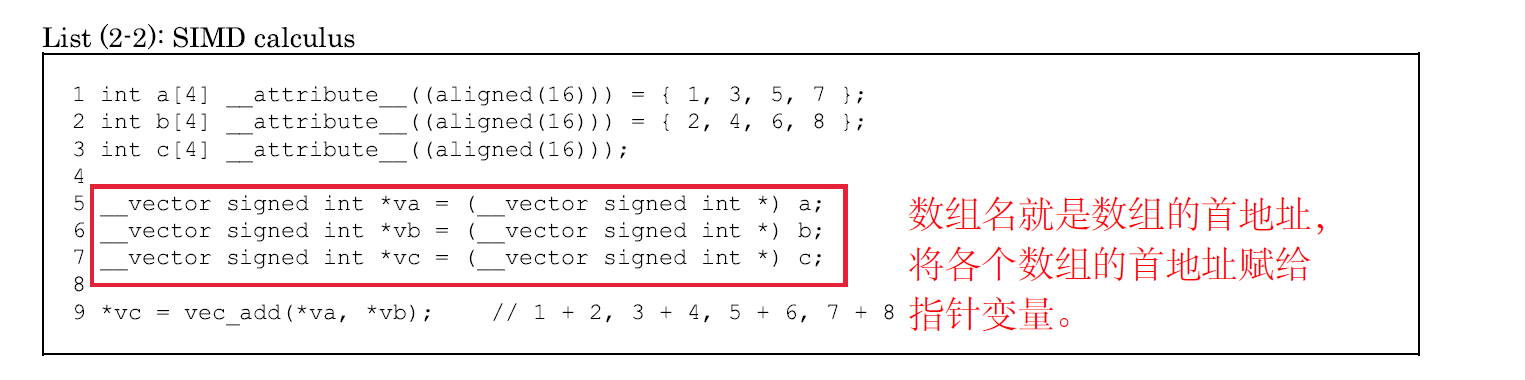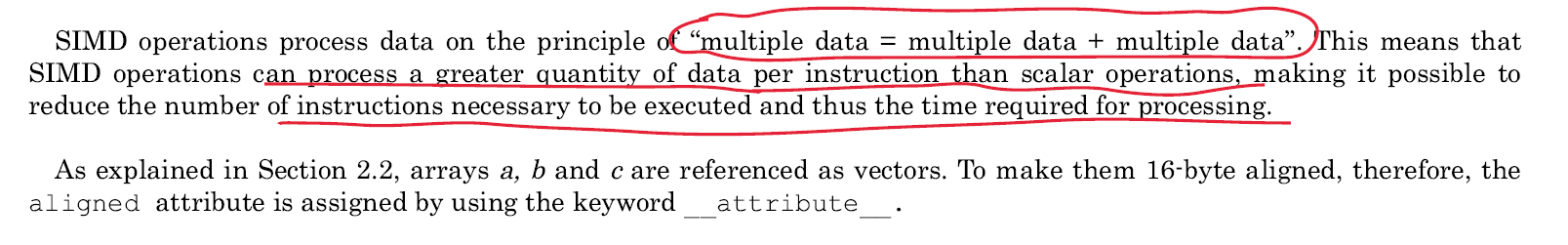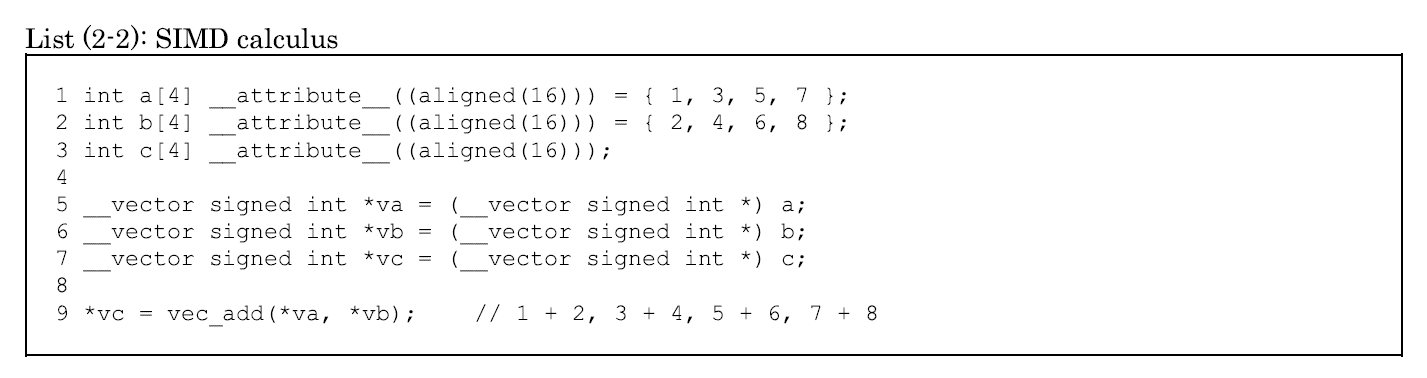​ c. 完整的加法例程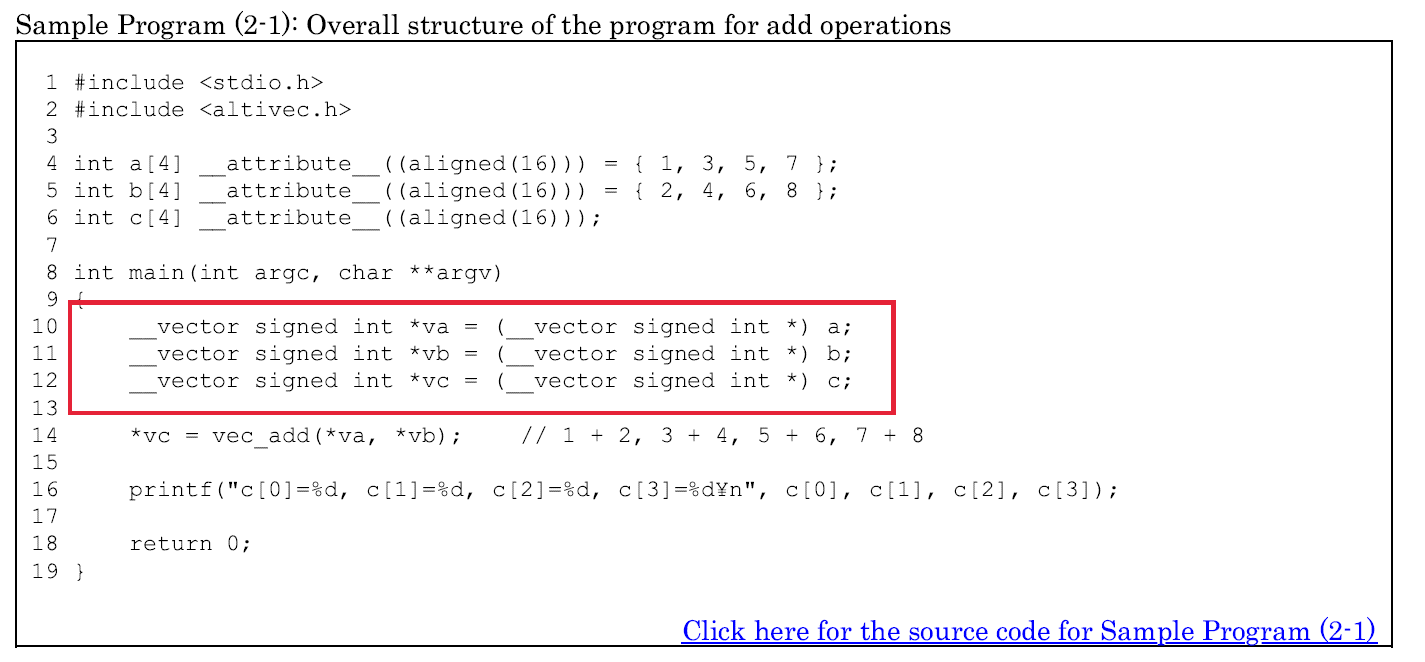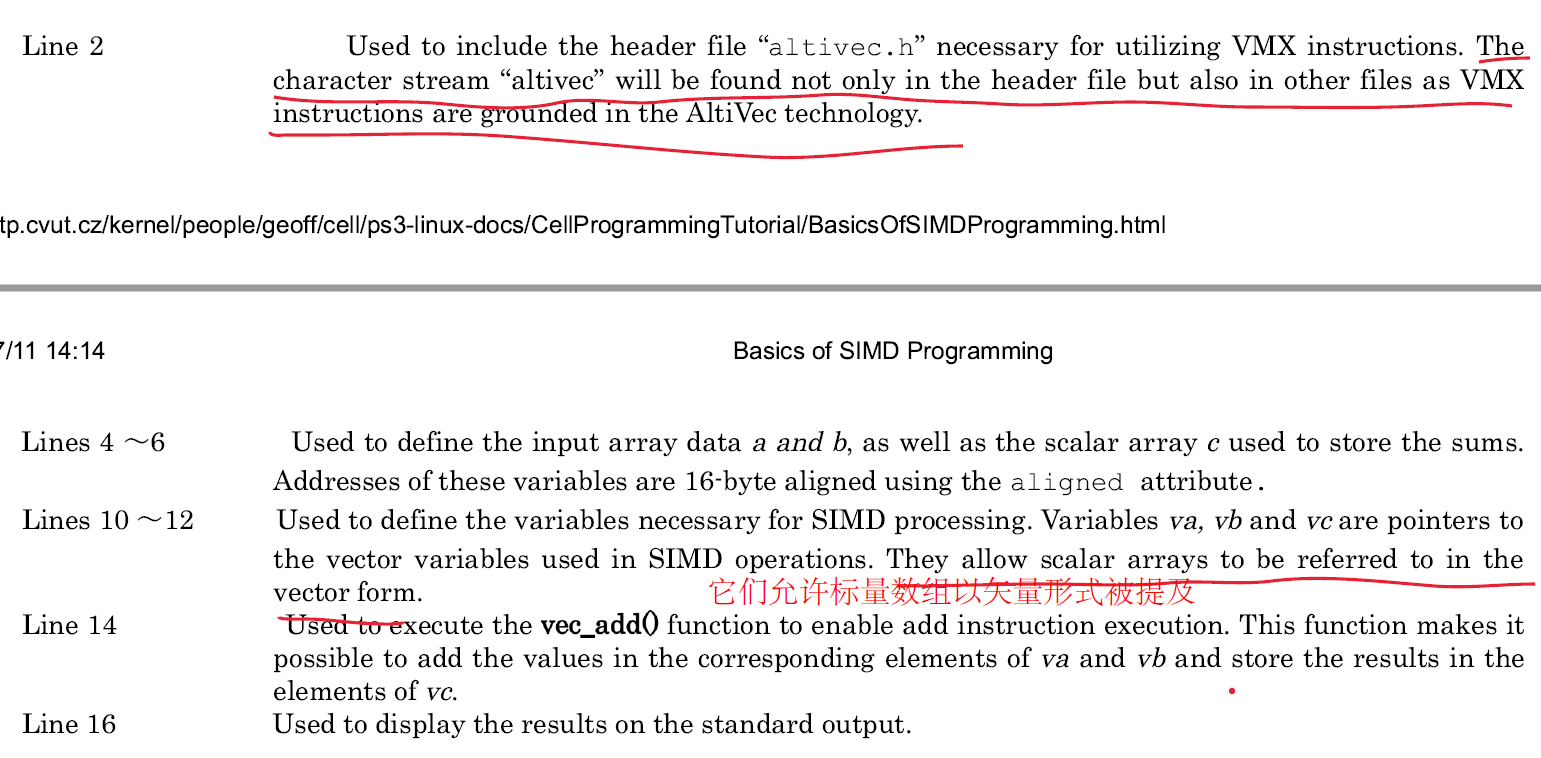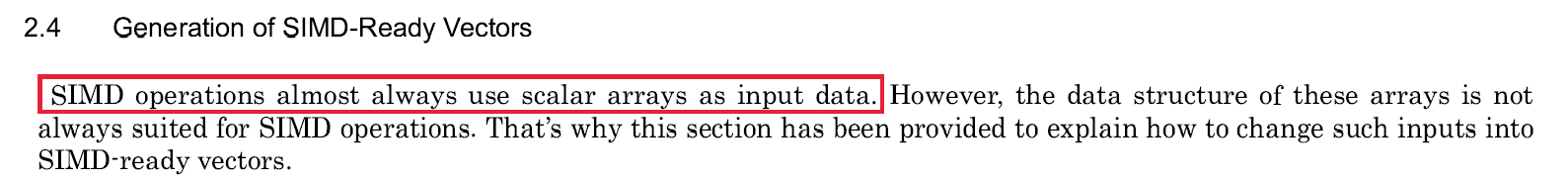1. 条件运算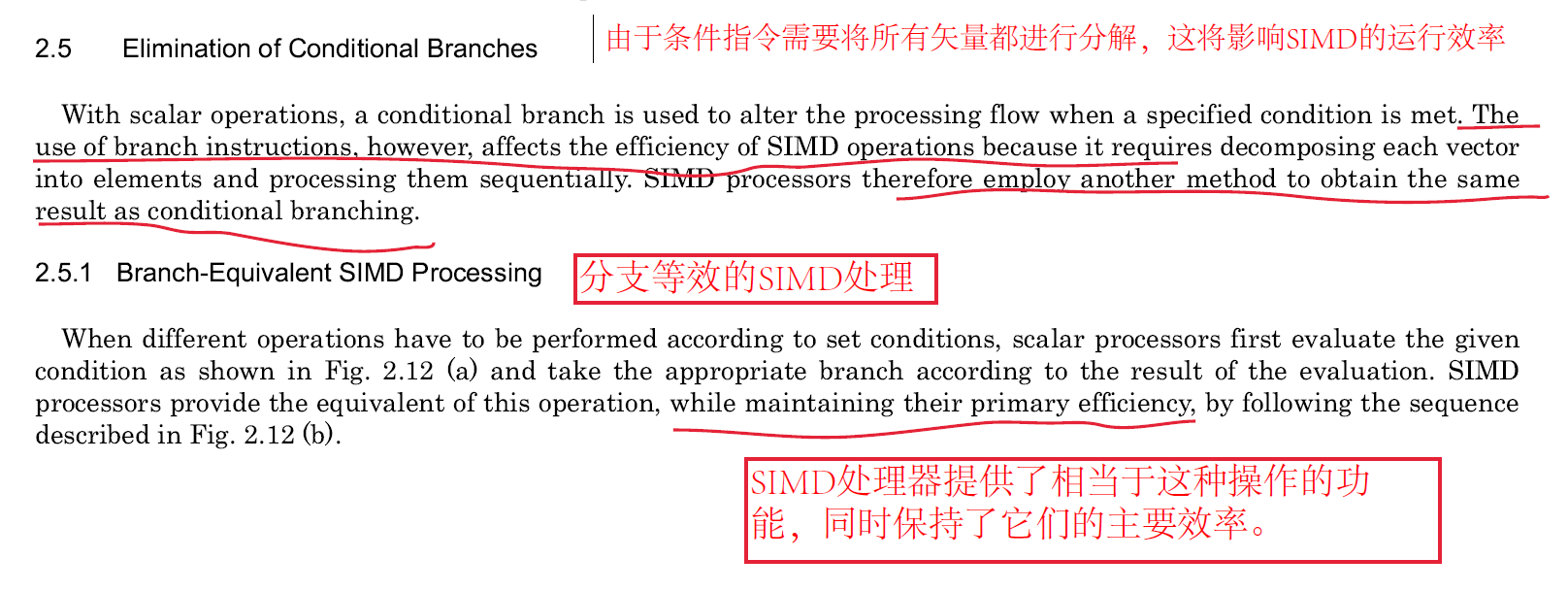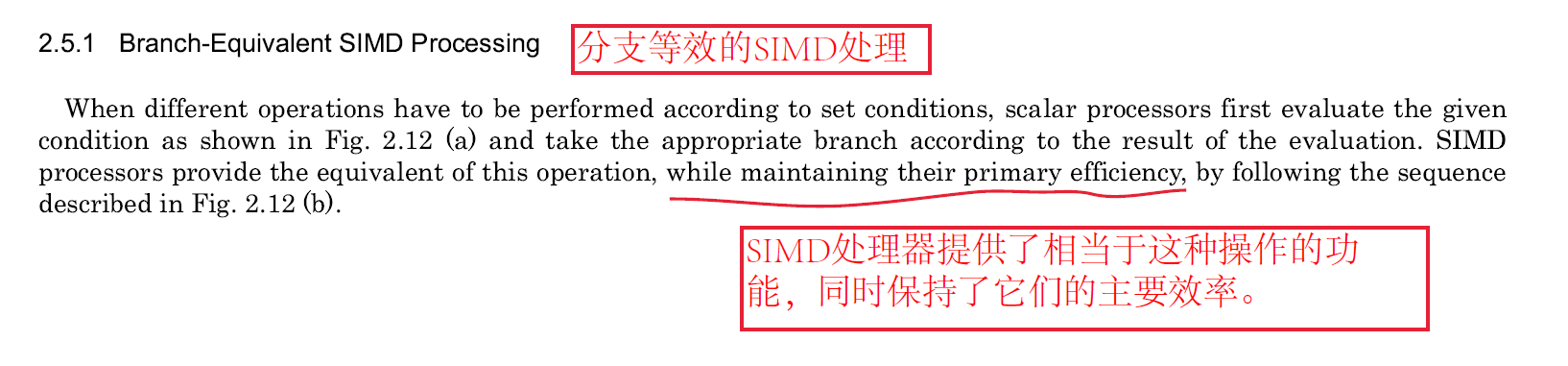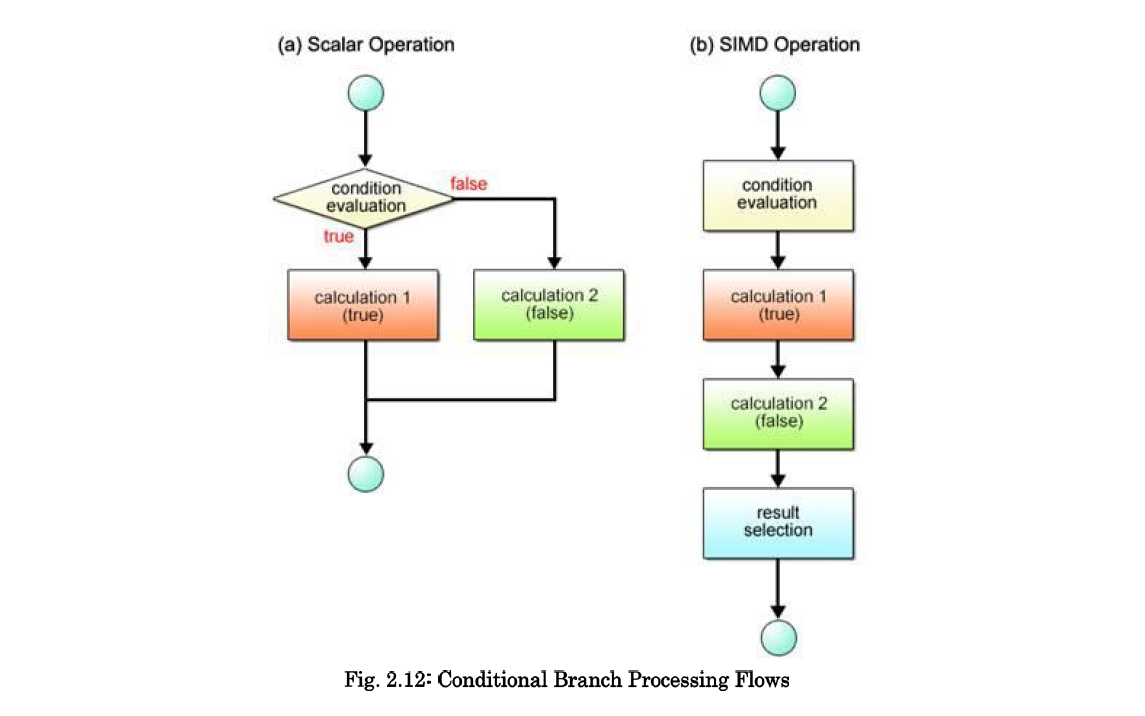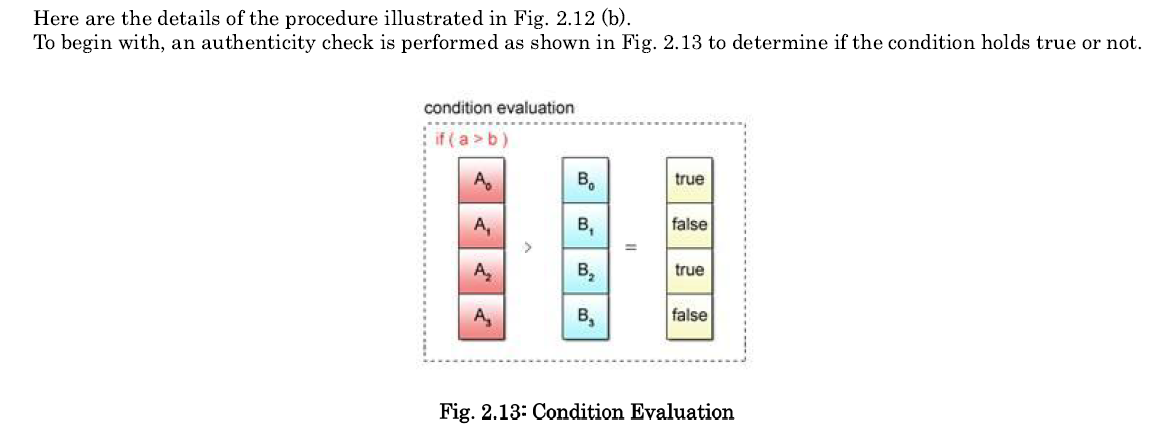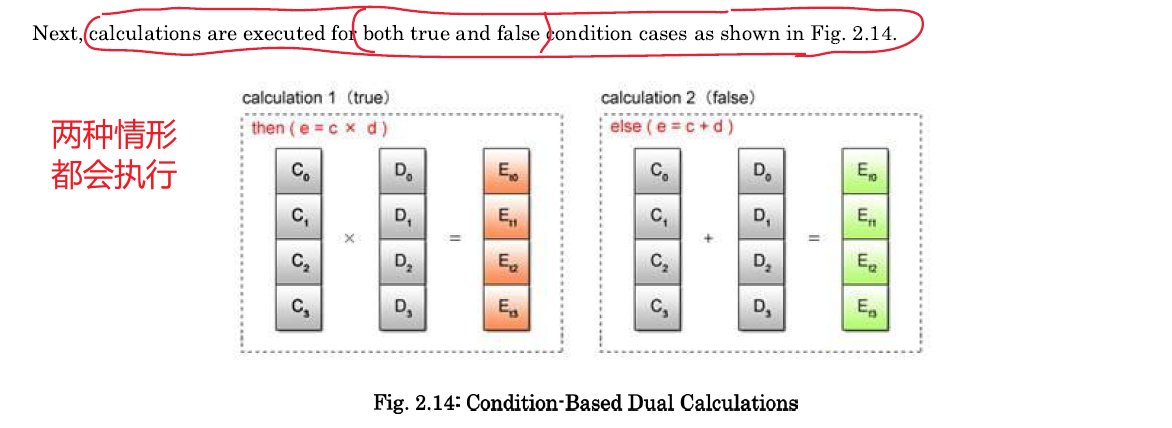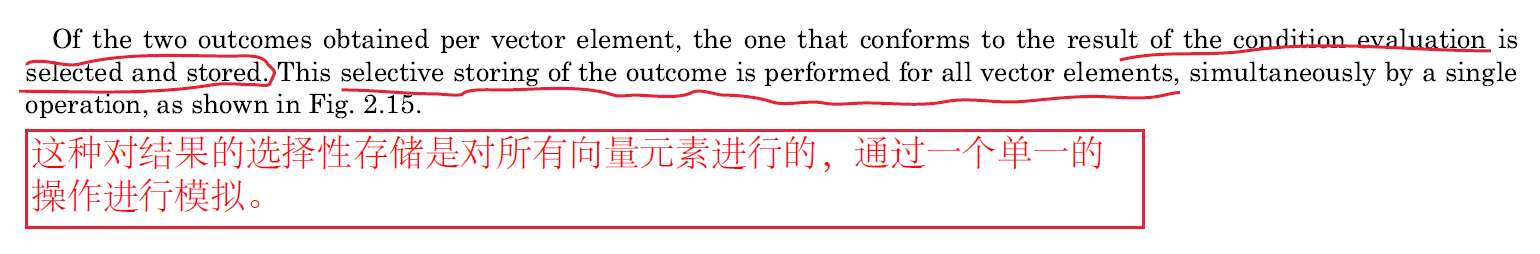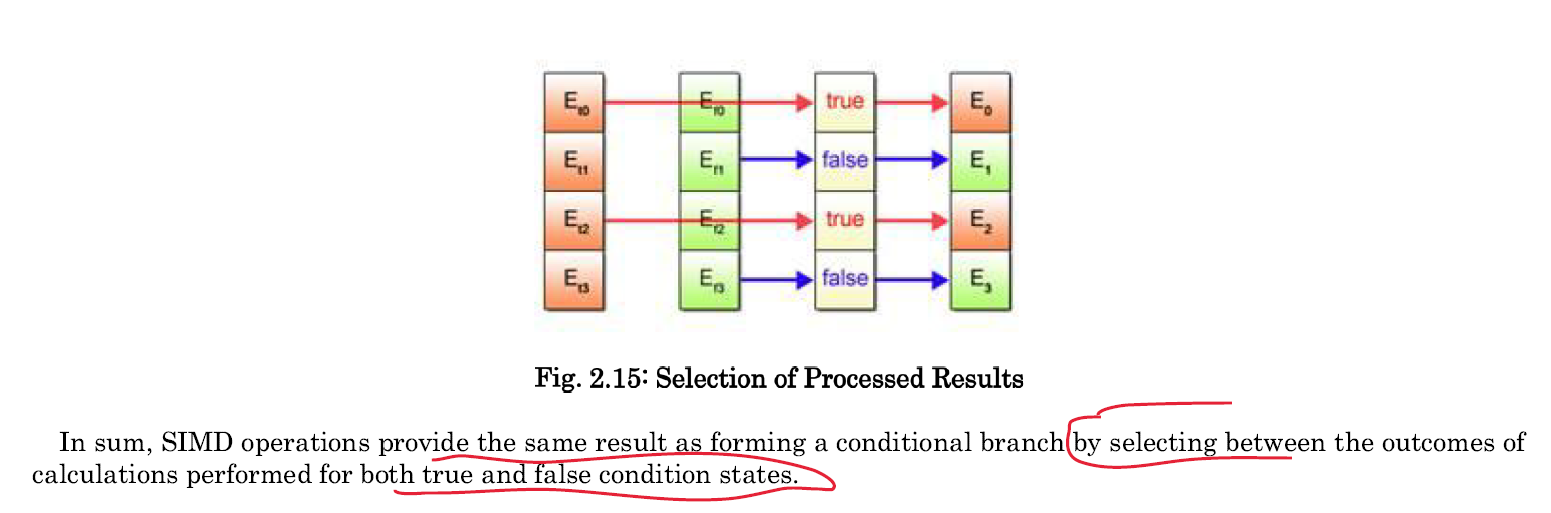## 五、寄存器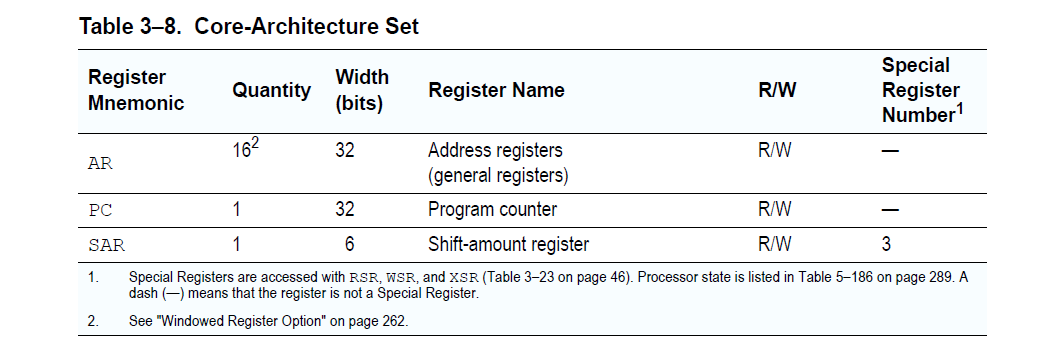### 5.2 移位和移位量寄存器（SAR）寄存器# Do more, know more, be more